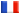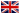# Séminaire de Cryptographie

## Bart de Smit### Standard models for finite fields

We give a deterministic polynomial time algorithm that on input two finite fields of the same cardinality produces an isomorphism between the two. Moreover, if for three finite fields of the same cardinality one applies the algorithm to the three pairs of fields then one obtains a commutative triangle. The algorithm depends on the definition of F_q as an actual field rather than a field up to isomorphism, which we call the standard model. This is joint work with Hendrik Lenstra.